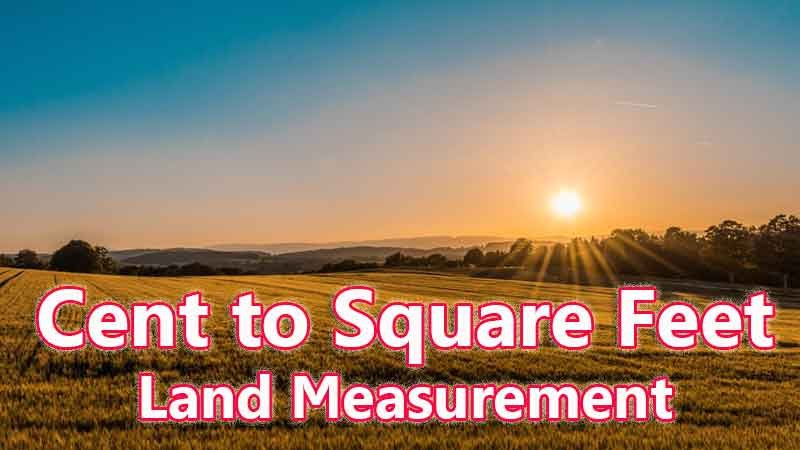# Cent To Square Feet Converter

Cent is a traditional land unit used to measure the land Area standardly. Here we are using the cent to square feet unit value conversion. And cent also comes under these land area units like Gonda, Kani, and square feet. In West Bengal, One cent is directly equivalent to 435.6 square feet.

Input Here

cent

Output

square feet

1 cent = 435.6 square feet
Rounded Value Is (1 cent = 436 square feet)

## Measurement

Cent means - a unit of land measurement. This tool provides great calculation over cent to square feet, 1 cent to square feet, 1 cent in square feet, 1 cent equal to and etc. To convert cent to square feet, then multiply the unit value by 435.6. Then, 1 cent * 435.6 = 435.6 square feet. Hence, 1 cent is equal to 435.6 square feet.

square feet also comes under this land measurement unit and we are comparing values between cent traditional unit with other side land measurement unit.

## Formula For cent to square feet Conversion(cent to square feet formula)

The base formula for this cent to square feet converter is

square feet = cent * 435.6

## How many square feet in a cent?

Step 1: To Convert 1 cent to square feet

Step 2: Applying formula square feet = cent * 435.6, (i.e) multiply the unit value by 435.6.

Step 3: Then, square feet = 1 * 435.6 = 435.6.

Step 4: Hence, 1 cent is equal to 435.6 square feet.Some cent to square feet Conversion Chart for your reference:

 1 cent = 435.6 square feet 2 cent = 871.2 square feet 3 cent = 1306.8000000000002 square feet 4 cent = 1742.4 square feet 5 cent = 2178 square feet 6 cent = 2613.6000000000004 square feet 7 cent = 3049.2000000000003 square feet 8 cent = 3484.8 square feet 9 cent = 3920.4 square feet 10 cent = 4356 square feet

The cent to square feet conversion chart is above listed for your reference. This chart, however, represents the simple math calculation involved in the cent to square feet convertion online.

For Example: How much is 1 cent to square feet

Solution:

= (cent * 435.6)

= (1 x 435.6)

= 435.6 square feet

For Example: How much is 120 cent in square feet

Solution:

= (cent * 435.6)

= (120 x 435.6)

= 52272 square feet

This formula provides an instant answer for all your questions / People also search:

• 1 cent to square feet
• 1 cent is equal to how many square feet
• Formula to convert cent to square feet online
• How to convert cent to square feet?
• How much square feet is equal to a one cent?
• How many square feet in cent?
• 1 cent equal to square feet